#### Algebraic Method for Combinational Logic, Physics tutorial

Introduction:

A logic circuit can be defined in the form of Boolean expression which, in turn, can be simplified using Boolean laws. A Boolean expression can also be transformed in the equivalent logic circuit. Whenever the logic circuit is explicitly stated by its truth table to give a fixed, invariant relationship between input and output, circuit is known as combinational circuit. The combinational circuit doesn't have memory. It always operates in accordance with its truth table in spite of of any previous input that may have been given to circuit. Boolean expression can be simplified in either of the two forms - (i) Sum of Product (SOP), and (ii) Product of Sum (POS). Object of simplification is to minimize number of variables or occurrences of the variable in expression. This signifies minimizing operation symbols and therefore the number of gates to be utilized in circuit. Several times, we get more than one simplified form of the expression, each being equivalent in number of gates and variables to be used. In final analysis, we shall utilize Minimum Sum of Product (MSP) form which is written without brackets. Consider reduced expression A (B + C) that is written in MSP form AB + AC. While reduced expression needs one AND gate and one OR gate, MSP expression needs one AND gates and one OR gate. Therefore in this situation MSP expression is not simplest. Fundamental rule is that expression should be (i) reduced as much as possible, and (ii) written without brackets. For simplification of Boolean expression, Boolean operations must be performed in given order:

i) Inversion of single variables.

ii) All operations with brackets.

iii) AND operations before OR operations.

iv) OR operations.

v) If the expression is with bar, then before inverting, carry out all operations.

E.g.: Find MSP expression for

Y = (A‾ + B‾)C‾ + AB‾

= (A‾ + B‾)C‾ + (A‾ + B‾) Using De Morgan's theorem Theorem

= (A‾ + B‾)(C‾ 1) Taking (A‾ + B‾) common

= (A‾ + B‾).1

= (A‾ + B‾)

= MSP expression

Getting the Truth Table from Boolean Expression:

Simple method of getting the truth table from Boolean expression is to substitute values of variables in each possible combination of values in expression. Carry out all logic operations and get result for each combination. For instance,

Y = AB + A (B + C) + B (B + C)

In this expression, say, A = 1, B = 0, and C = 0, then

Y = 1 . 0 + 1 . (0 + 0) + 0 (0 + 0)

= 0 + 1 . 0 + 0 . 0

= 0 + 0 + 0

= 0

Likewise, determine Y for all combinations of values for A, B, and C.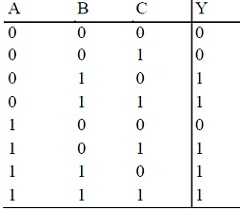Getting Boolean Expression from a Truth Table:

Consider truth table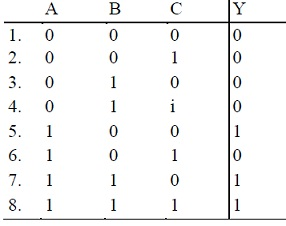Note, that entries 5, 7, and 8 give the logic 1 to operation whereas all other entries provide logic 0. To get Boolean expression, write product term for each entry that give a logic 1, and then assemble operations by connecting all products with logic

Entry 5: Y = 1 for A = 1, B = 0, C = 0

= AB‾C‾

As output of AND gate will be 1 only if all inputs are 1. Likewise

Entry 7: Y = 1 for A = 1, B = 1, C = 0 = ABC‾

Entry 8: Y = 1 for A = 1, B = 1, C = 1 = ABC

Now join all three products with OR logic. Therefore Y = AB‾C‾ + ABC‾ + ABC (Sum of Product), that can be simplified as

Y = AB‾C‾ + AB(C‾ + C)

= AB‾C‾ + AB

= A(B‾C‾ + B)

= A(B + C‾)

= AB + AC‾

Exclusive- OR (XOR) Gate:

XOR gate gives the high output (i.e. 1) when the odd number of inputs is high. Two-input exclusive - OR gate has its output 1 if one of two inputs is 1 and other is 0, and if both inputs are same then output is 0.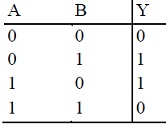Its Boolean expression is t is Y = A‾B + AB‾. This expression is in MSP form as it can't be simplified further. Therefore Y is output of OR gate inputs to which are A‾B and AB‾, which in turn are outputs of two AND gates. The XOR operation is expressed by ⊕.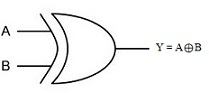Exclusive -NOR (XNOR) Gate: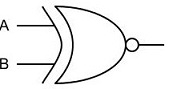Exclusive-NOR gate has its output 1 if both inputs are same, and if both inputs are different then output is 0. Truth table of XNOR gate is: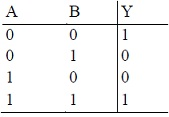Its Boolean expression is:

Y = A‾B‾ + AB

Addition of Two One Bit Binary Numbers (Half Adder):

This application has two outputs, one for sum and another for carry. Thus, we have to get two Boolean expressions for two outputs.

Truth table for half adder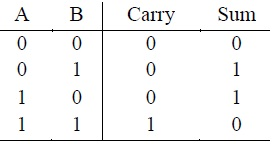Expression for carry is

Carry = AB that is, it is the output of an AND gate.

The expression for sum is

Sum = A‾B + AB‾

It is the output of XOR gate. These two circuits are connected together as shown. This circuit is known as half adder and its symbol is given below: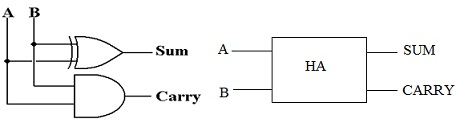Addition of Three One Bit Binary Numbers (Full Adder):

Full adder can add three single-bit binary numbers. Binary addition three single-bit binary numbers is as follows:

Right hand bits of the additions represent sum and left hand bits represent the carry.

The eight possible combinations of three single-bit binary numbers can be presented in form of truth table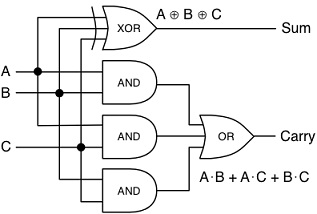To design logic circuit for full adder, Boolean expressions have to be written and simplified in MSP form for both sum and carry, that are as follows:

Sum = A‾B‾C + A‾BC‾ + AB‾C‾ + ABC ABC + ABC + ABC + ABC

= A‾(B‾C + BC‾) + A(B‾C‾ + BC)

= A‾(B ⊕ C) + A[(B ⊕ C)‾]

= A‾X + AX‾ where X = B ⊕C

= A⊕ B ⊕C

= MSP expression.

This is the output of a 3-input XOR gate.

Carry = A‾BC + AB‾C + ABC‾ + ABC

= BC(A‾ + A) + AB‾C + ABC‾ BC(A + A) + ABC + ABC

= BC + AB‾C + ABC‾

= C(B + AB‾) + ABC‾

= C(B + A) + ABC‾

= BC + AC + ABC‾

= BC + A(C + BC‾)

= BC + A(C + B)

= BC + AC + AB = MSP expression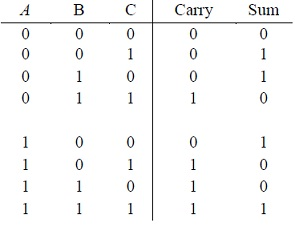A computer or digital circuit can add only two binary numbers at a time. If the digital circuit has to add more than two binary numbers, the circuit will add first two binary numbers and to sum of these two numbers it will add third binary number, and so on. But while adding two bits a carry is liable to appear.

Designing Circuits Using NAND Gates Only:

Quite often it is needed that only NAND gates must be utilized in designing digital circuits. NAND gate being universal can be utilized to realize AND, OR and NOT gates. Thus, wherever these gates are appearing, equivalent NAND circuit is utilized. The realization of AND, OR and NOT gates from NAND gates.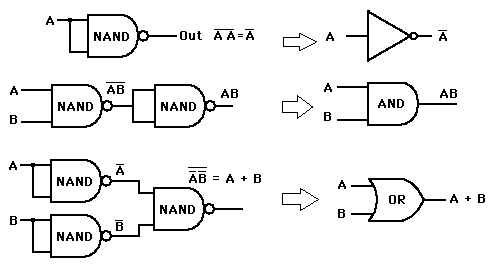Tutorsglobe: A way to secure high grade in your curriculum (Online Tutoring)

Expand your confidence, grow study skills and improve your grades.

Since 2009, Tutorsglobe has proactively helped millions of students to get better grades in school, college or university and score well in competitive tests with live, one-on-one online tutoring.

Using an advanced developed tutoring system providing little or no wait time, the students are connected on-demand with a tutor at www.tutorsglobe.com. Students work one-on-one, in real-time with a tutor, communicating and studying using a virtual whiteboard technology.  Scientific and mathematical notation, symbols, geometric figures, graphing and freehand drawing can be rendered quickly and easily in the advanced whiteboard.

Free to know our price and packages for online physics tutoring. Chat with us or submit request at info@tutorsglobe.com